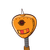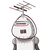# If (Sec A + tan A ) (SecB + tanB) (Sec C + tanC) = (Sec A -tanA) (Sec B – tan B) (Sec C – tan C ) . Prove that each of the side is

If (Sec A + tan A ) (SecB + tanB) (Sec C + tanC) = (Sec A -tanA) (Sec B – tan B) (Sec C – tan C ) . Prove that each of the side is equal to ±1 .

❒ Best Of Luck !!
❒Spammers Stay Away !!​

### 2 thoughts on “If (Sec A + tan A ) (SecB + tanB) (Sec C + tanC) = (Sec A -tanA) (Sec B – tan B) (Sec C – tan C ) . Prove that each of the side is”

1.★GIVEN:-

\sf{(Sec A + tan A ) (SecB + tanB) (Sec C + tanC) = (Sec A -tanA) (Sec B – tan B) (Sec C – tan C ) }(SecA+tanA)(SecB+tanB)(SecC+tanC)=(SecA−tanA)(SecB−tanB)(SecC−tanC)

★TO PROVE:-

each of the side is equal to ±1

★FORMULA USED:-

\sf{Sec^2x – Tan ^2x =1}Sec

2

x−Tan

2

x=1

★LET:-

(Sec A + tan A ) (SecB + tanB) (Sec C + tanC)——–(1)

(Sec A -tanA) (Sec B – tan B) (Sec C – tan C )————–(2)

★SOLUTION:-

Multiply Eqs (1) and (2) we get,

\sf{ (Sec^2A – Tan^2A)(Sec^2B – Tan ^2 B )( Sec ^2 C – Tan ^2 C ) = 1}(Sec

2

A−Tan

2

A)(Sec

2

B−Tan

2

B)(Sec

2

C−Tan

2

C)=1

\sf{[(Sec A – Tan A)(Sec B – Tan B )(Sec C – Tan C )]^2 = 1}[(SecA−TanA)(SecB−TanB)(SecC−TanC)]

2

=1

\sf{(Sec A – Tan A)(Sec B – Tan B )(Sec C – Tan C ) = \pm \: 1}(SecA−TanA)(SecB−TanB)(SecC−TanC)=±1

HENCE, each of the side is equal to ±1

2.